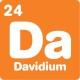# Blog

## 1936 brainteaser

By

,

1936 is a square number. That means you can make it by multiplying a whole number by itself:
1936 = 44 x 44

I recently did some maths to get 1936:

• I started with a single digit square number
• I added one digit to it to get another square
• Then I added another digit to get another square
• Then I added another digit and I got 1936, which is also a square!

Can you work out all the squares I made?There are lots of ways to solve this puzzle – here’s one:

• The single digit square must have been 1 or 9 – there is no 4 in 1936.
• Similarly, the two digit square must have been 16 or 36.
• From these two, you can tell that my squares must have been 1, 16, ???, 1936.
• A bit of mucking about on a calculator reveals that 14 x 14 = 196. This fills the gap: 1, 16, 196, 1936.

If you like bending the rules a bit, you can also have: 9, 36, 361, 1936, as long as you’re okay with flipping the 9 upside down!

For more brainteasers and puzzles for kids, subscribe to Double Helix magazine!Categories:

## 2 responses

1.David Hamon

No wonder that I could never understand maths at school nor now! I cannot understand what your exercise involved. Nor did the answer make this any clearer. How did the fact that there is no 4 in 1936 establish that the number must be 1 or 9, or similarly that the two digit square must have been 16 or 36. What then leads to the next line or to the last line 1, 16, 196, 1936. What is this series anyway and what would make you think that this is what you had to arrive at? This all seems to require a fairly intimate knowledge of maths before you can even get started and/or understand the answer. You have to understand to be able to learn. My maths teacher used to assume that just because he said something that you must understand it! I didn’t!

1.David

I’m sorry our answer wasn’t easy to follow!

Actually, I think the difficulty is the question itself. I wanted you to pick a 1 digit square, a 2 digit square, a three digit square and a four digit square so that each was just the last with an extra digit added in. Then I gave the last of those numbers.

With that in mind, the one digit square must be 1, 9, 3 or 6. since 3 and 6 aren’t squares, it’s gotta be one of those two.

The only two digit squares you can make with 1,9,3,6 are 16 and 36, and the only one of those you can make by adding a digit to 1 or 9 is 16.

It’s a bit confusing and I’m sorry that you had trouble. We’ll make the next one a bit clearer, I promise!

This site uses Akismet to reduce spam. Learn how your comment data is processed.

By posting a comment you are agreeing to the Double Helix commenting guidelines.